# Hydrostatic force

What hydrostatic force is applied to an area of 30 cm2 in water at a depth of 20 m? (Water density is 1000 kg/m3)

Result

F =  588.6 N

#### Solution:Leave us a comment of example and its solution (i.e. if it is still somewhat unclear...):Be the first to comment!#### To solve this verbal math problem are needed these knowledge from mathematics:

Do you want to convert area units? Do you want to convert mass units?

## Next similar examples:

1. Air pressure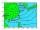The meteorological station measured the air pressure of 1060 hPa (hectopascals). In addition to measuring instruments, there is a residential house that has a flat roof with dimensions of 10 m and 28 m. What pressure force in MN (meganewtons) force the aiWhat overload in g (g-force) has passed the pilot if he accelerated from 0 to 600 km/h in 3 seconds?
3. Copper wire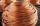What is the weight of 1000 m copper wire with a diameter of 5 mm when rezistivity p = 8.8 g / cm3?
4. Theorem proveWe want to prove the sentence: If the natural number n is divisible by six, then n is divisible by three. From what assumption we started?
5. ResistanceA resistor having an electrical resistance of 1.5 k ohms passes an electrical current of 0.1 A. Calculate what voltage is between the terminals of the resistor.
6. Average speed 4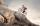Starting at home Tony traveled uphill to the store for 45 minutes at 8 miles per hour. he then traveled back home on the same path at a speed of 24 miles per hour. what is his average speed for the entire trip?
7. PotatoesFor three days the store sold 1400 kg of potatoes. The first day they sold 100 kilograms of potatoes less than the second day, the third-day three-fifths of what they sold the first day. How many kgs of potatoes sold every day?
8. Barrel 4Barrel of water weighs 63 kg. After off 75% water, the weight of the barrel with water is 21 kg. How many kg weigh empty barrel and how many kgs water in it?
9. Mass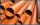The thickness of a metallic tube is 1cm and its outer radius is 11cm. Find the mass of such 1m long tube, if the density of the metal is 7.5g per cubic cm.
10. Potatoes bags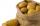I have three bags with 21 kg potatoes. The first bag is 5.5 kg more than in the second bag and the third is 0.5 kg more than in the second bag. Determine how many kgs of potatoes is in each bag.
11. Functions f,gFind g(1) if g(x) = 3x - x2 Find f(5) if f(x) = x + 1/2
12. Nineteenth memberFind the nineteenth member of the arithmetic sequence: a1=33 d=5 find a19
13. Three friendsDanica, Lenka and Dalibor have altogether 96 kg. Lenka weighs 75% more than Dalibor and Danica weighs 6 kg more than Dalibor. Determine the weight of Danice, Lenka and Dalibor.
14. Volleyball8 girls wants to play volleyball against boys. On the field at one time can be six players per team. How many initial teams of this girls may trainer to choose?
15. TreesA certain species of tree grows an average of 0.5 cm per week. Write an equation for the sequence that represents the weekly height of this tree in centimeters if the measurements begin when the tree is 200 centimeters tall.
16. PowersExpress the expression ? as the n-th power of the base 10.
17. Trio weight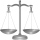Adelka, Barunka, and Cecilka are weight in pairs. Adelka with Barunka weighs 98 kg, Barunka with Cecilka 90 kg and Adelka with Cecilka 92 kg. How much does each of them weigh?